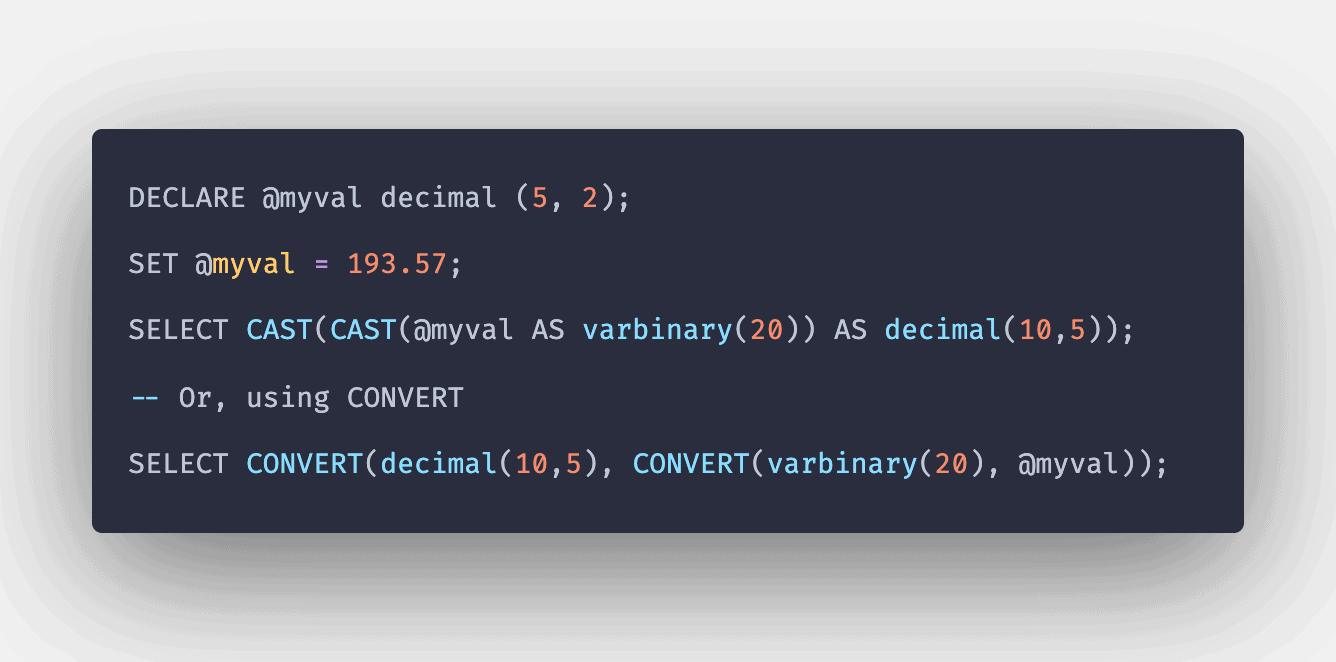# SQL CONVERT Function: Complete Guide

0
0SQL CONVERT() function converts the value (of any type) into a specified datatype.

## SQL CONVERT

SQL CONVERT() is a built-in function that is used for converting an expression from one data type to another data type. Here, if the conversion takes place, then a value with a specified conversion will be returned. Otherwise, the function will return an error.

### Syntax

```CONVERT ( target_type [ ( length ) ] , expression [ , style ] )
```

### Parameters

1. Target_type: The data type to which the expression will be converted.
2. Length: It is entirely optional. It signifies the length of the resulting data type for expression. It defaults up to 30.
3. Expression: The value to be converted to another datatype.
4. Style: It is an optional integer that determines the format of the converted expression.

#### Note

1. The result is truncated when the expression is converted to integer datatype when converted from float or integer.
2. For other conversions, the value is rounded.

### Query 1

```SELECT CONVERT (INT, 10.85);
```

`10`

#### Explanation

As the expression is converted from float to integer. So, the result here is truncated.

### Query 2

```SELECT CONVERT (float, 10.85);
```

```10.85
```

#### Explanation

As the expression is converted to float datatype. So, the result here is not truncated, and the original expression was returned, which was already in the float.

### Query 3

```SELECT CONVERT (varchar, 15.6);
```

#### Output

```'15.6'
```

#### Explanation

Here, the floating expression was converted to a character data type.

### Query 4

```SELECT CONVERT (float, ‘15.6’);
```

```15.6
```

#### Explanation

Here, the character data type is converted to a floating-point value.

### Query 5

```SELECT CONVERT (datetime, '2020-02-26');
```

#### Output

```'2020-02-26 00:00:00.000'
```

#### Explanation

Here, the string expression is converted to a datetime expression.

### Query 6

```SELECT CONVERT (varchar, '02/26/2020', 101);
```

#### Output

```'02/26/2020'
```

#### Explanation

The Convert() function converted the current date to varchar datatype with the specified style.